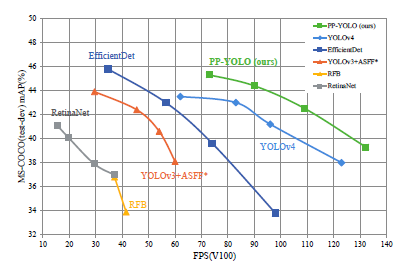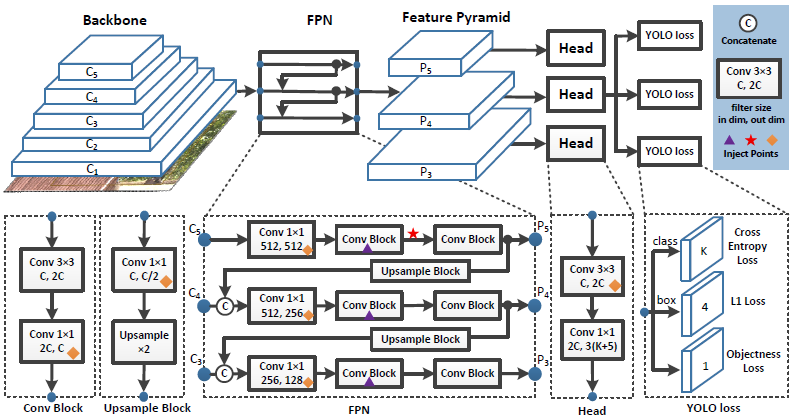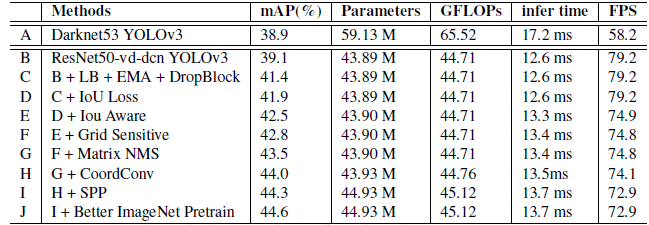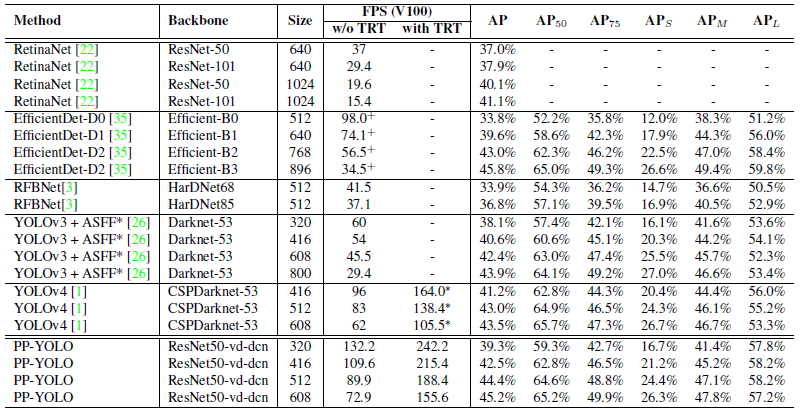# Review — PP-YOLO: An Effective and Efficient Implementation of Object Detector

## PP-YOLO, Outperforms EfficientDet & YOLOv4PP-YOLO runs faster than YOLOv4 and improves mAP from 43.5% to 45.2%

PP-YOLO: An Effective and Efficient Implementation of Object Detector
PP-YOLO
, by Baidu Inc.,
2020 arXiv v3, Over 90 Citations (Sik-Ho Tsang @ Medium)
Object Detection, YOLO series

• PP-YOLO is proposed, which is a new object detector based on YOLOv3.
• It combines various existing tricks that almost not increase the number of model parameters and FLOPs, to achieve the goal of improving the accuracy of detector as much as possible while ensuring that the speed is almost unchanged.
• PP means PaddlePaddle framework by Baidu.

# Outline

1. Architecture
2. Selection of Tricks
3. Experimental Results

# 1. ArchitectureThe network architecture of YOLOv3 and inject points for PP-YOLO

## 1.1. Backbone

• DarkNet-53 is first applied to extract feature maps at different scales.
• DarkNet-53 is replaced with ResNet50-vd in PP-YOLO.
• Some convolutional layers in ResNet50-vd are replaced with deformable convolutional layers, as originated in DCN. In order to balance the efficiency and effectiveness, we only replace 3×3 convolution layers in the last stage with DCNs.
• This modified backbone is named as ResNet50-vd-dcn, and the output of stage 3, 4 and 5 as C3, C4, C5.

## 1.2. Detection Neck

• Feature maps C3, C4, C5 are input to the FPN module.
• The output feature maps of pyramid level l as Pl, where l=3, 4, 5.

## 1.3. Detection Head

• It consists of two convolutional layers. A 3×3 convolutional followed by an 1×1 convolutional layer is adopt to get the final predictions.
• The output channel of each final prediction is 3(K+5). Because each prediction position has 3 anchors. For each anchor, the first K channels are the prediction of probability for K classes. The following 4 channels are the prediction for bounding box localization. The last channel is the prediction of objectness score.
• For classification and localization, cross entropy loss and L1 loss is adopt correspondingly. An objectness loss is applied to supervise objectness score, as in YOLOv3, which is used to identify whether is there an object or not.

# 2. Selection of Tricks

## 2.1. Larger Batch Size

• The training batch size is increased from 64 to 192, and the training schedule and learning rate are adjusted accordingly.

## 2.2. Exponential Moving Average (EMA)

• EMA computes the moving averages of trained parameters using exponential decay:

## 2.4. IoU Loss

• In YOLOv3, L1 loss is adopted for bounding box regression. It is not tailored to the mAP evaluation metric.
• Different from YOLOv4, the L1-loss is not replaced with IoU loss directly, but it is added using another branch to calculate IoU loss.

## 2.5. IoU Aware

• In YOLOv3, the classification probability and objectness score is multiplied as the final detection confidence, which do not consider the localization accuracy.
• To solve this problem, an IoU prediction branch is added to measure the accuracy of localization. During training, IoU aware loss is adopt to training the IoU prediction branch.
• During inference, the predicted IoU is multiplied by the classification probability and objectiveness score to compute the final detection confidence, which is more correlated with the localization accuracy.
• The final detection confidence is then used as the input of the subsequent NMS.

## 2.6. Grid Sensitive

• Grid Sensitive is an effective trick introduced by YOLOv4.
• In YOLOv3, the coordinate of the bounding box center x and y is decoded by:
• This makes it difficult to predict the centres of bounding boxes that just located on the grid boundary. The above equation is modified as below:
• where α is set to 1.05 in this paper. This makes it easier for the model to predict bounding box center exactly located on the grid boundary.

## 2.7. Matrix NMS

• Matrix NMS is motivated by Soft-NMS, which decays the other detection scores as amonotonic decreasing function of their overlaps.
• Matrix NMS is implemented in a parallel manner, which is faster than traditional NMS.

## 2.8. CoordConv

• Inly the 1×1 convolution layer in FPN and the first convolution layer in detection head is replaced with CoordConv.

## 2.9. Spatial Pyramid Pooling (SPP)

• The SPP, originated in SPPNet, only applied on the top feature map as shown in the above figure with “star” mark.
• Around 2% additional parameters and 1% extra FLOPs are introduced.

## 2.10 Better Pretrain Model

• The distilled ResNet50-vd model is used as the pretrain model.

# 3. Experimental Results

## 3.1. Ablation StudyThe ablation study of tricks on the MS-COCO minival split
• The above table shows the incremental improvement for each component.

## 3.2. SOTA ComparisonComparison of the speed and accuracy of different object detectors on the MS-COCO (test-dev 2017)
• PP-YOLO has certain advantages in speed and accuracy.
• Compared with YOLOv4, PP-YOLO can increase the mAP on COCO from 43.5% to 45.2% with FPS improved from 62 to 72.9. It is worth noticing that tensorRT accelerates the PP-YOLO model more obviously.
• The relative improvement of PP-YOLO (around 100%) is larger than YOLOv4 (around 70%). It is mainly because tensorRT optimizes for ResNet model better than Darknet.

PP-YOLO results have advantages in the balance of speed and accuracy compared with other detectors.

PP-YOLO has been extended as PP-YOLOv2 and PPYOLOE, and PPYOLOE has been used for comparison in YOLOv7.

## Reference

[2020 arXiv v3] [PP-YOLO]
PP-YOLO: An Effective and Efficient Implementation of Object Detector

## Object Detection

2020 [EfficientDet] [CSPNet] [YOLOv4] [SpineNet] [DETR] [Mish] [PP-YOLO] 2021 [Scaled-YOLOv4] [PVT, PVTv1] [Deformable DETR] 2022 [PVTv2]

--

--